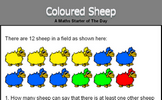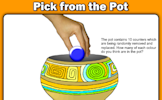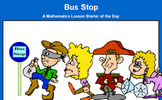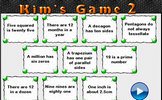# Sets and probability

### Term 3 starting in week 9 :: Estimated time: 2 weeks

• Identify and represent sets
• Interpret and create Venn diagrams
• Understand and use the intersection of sets
• Understand and use the union of sets
• Know and use the vocabulary of probability
• Generate sample spaces for single events
• Calculate the probability of a single event
• Understand and use the probability scale
• Know that the sum of probabilities of all possible outcomes is 1

For higher-attaining pupils:

• Understand and use the complement of a set

This page should remember your ticks from one visit to the next for a period of time. It does this by using Local Storage so the information is saved only on the computer you are working on right now.

## Lesson Starters

Here are some suggestions for whole-class, projectable resources which can be used at the beginnings of each lesson in this block.

### 1st Lesson#### Venn Diagram

Arrange numbers on the Venn Diagram according to their properties.

### 2nd Lesson#### Coloured Sheep

What is the probability of picking a red sheep from the sheep in the field?

### 3rd Lesson#### Pick From The Pot

The pot contains 10 counters which are being randomly removed and replaced. How many of each colour do you think are in the pot?

### 4th Lesson#### Ice Cream

How many different ice cream cones can be made by choosing two scoops from six flavours?

### 5th Lesson#### Bus Stop

How many different ways can four people stand in line?

### 6th Lesson#### Kim's Game 2

This is the Maths version of the traditional memory game. Memorise 10 mathematical facts then recall them as they disappear from view.

Some of the Starters above are to reinforce concepts learnt, others are to introduce new ideas while others are on unrelated topics designed for retrieval practice or and opportunity to develop problem-solving skills.

White Rose ResourcesEnd of block assessments provide a quick progress check at the end of each block of learning to make sure students have understood the content covered. This Scheme of Learning was produced by White Rose Maths and is used here with permission granted on 30th June 2021.For All: